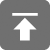# 工学1号馆

home

## 并查集(Disjoint Set)

Wu Yudong    October 18, 2016     Data Structure   475

## 定义

• 合并两个集合
• 查找某元素属于哪个集合## 算法实现

find1(x)
{
return set[x];
}

Merge1(a,b)
{
i = min(a,b);
j = max(a,b);
for (k = 1; k <= N; k++) {
if (set[k] == j)
set[k] = i;
}
}

## 算法的优化

### 使用树结构

• set[i] = i , 则i表示本集合，并是集合对应树的根
• set[i] = j, j<>i, 则 j 是 i 的父节点.find2(x)
{
r = x;
while (set[r] != r)
r = set[r];
return r;
}

merge2(a, b)
{
if (a<b)
set[b] = a;
else
set[a] = b;
}

### 优化–避免最坏情况

max(h1,h2), if h1<>h2.
h1+1, if h1=h2.

find2(x)
{
r = x;
while (set[r] != r)
r = set[r];
return r;
}

merge3(a,b)
{
if (height(a) == height(b)) {
height(a) = height(a) + 1;
set[b] = a;
} else if (height(a) < height(b)) {
set[a] = b;
} else {
set[b] = a;
}
}

### 进一步优化–路径压缩

• 第一步，找到根结点
• 第二步，修改查找路径上的所有节点，将它们都指向根结点

find3(x)
{
r = x;
while (set[r]  !=  r) //循环结束，则找到根节点
r = set[r];
i = x;
while (i != r) //本循环修改查找路径中所有节点
{
j = set[i];
set[i] = r;
i = j;
}
}